# Double and triple

Find the number to which it is true:
its double is 5 less than its triple.

x =  5

### Step-by-step explanation:

2x = 3x-5

2•x = 3•x-5

x = 5

Our simple equation calculator calculates it.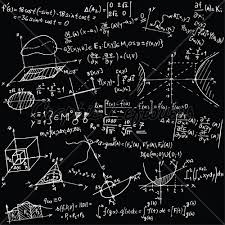Did you find an error or inaccuracy? Feel free to write us. Thank you!Tips to related online calculators
Do you have a linear equation or system of equations and looking for its solution? Or do you have a quadratic equation?

## Related math problems and questions:

• Unknown number 6Determine the unknown number, which is by 1.5 greater than its fourth.
• NumberI think the number. If I add to its third seven I get same as when to its quarter add 8. Which is the number?
• Unknown numberFind the unknown number equal to a quarter of a fifth of a number, which is by 152 more than an unknown number.
• Unknown numberI think the number. I'll reduce it to its one-third. The result is then increased by one-third, and I get the number 12.
• Perpetrator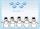The perpetrator is a number that is smaller than the number 80. It is a multiple of five and it is odd. If we added up the tens and ones of this number we get number 8.
• Number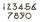What number do I think? The third is 6, and a half is 2 more than its quarter.
• Unknown number 24I think the number: a - is the same as the square area that has the 12th circumference. What is this number? b - its half is 7 times bigger than its quarter. Is this the number?
• TimeageSeven times my age is 8, less than the largest two-digit number. How old am I?
• Unknown number 6Determine x if 1/6 of x is equal to 2/5 of the number 24.
• Unknown numberFind an unknown number of which 1/5 is 40 greater than one-tenth of that number.
• 15 numberWhat number is smaller (greater) by 15 than its half?
• NumberDetermine unknown number if you know that difference between five times and triple of number is 42.
• Brackets 2Add parenthesis to make true: 5-2×6-4+2=5
• Rounding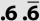Double round number 727, first to tens, then to hundreds. (double rounding)
• Unknown number 5I think of an unknown number. If we enlarge it five times then subtract 3 and the result decreases by 75% we get one greater than the number. What number am I thinking of?
• Roll the diceWhat is the probability that if we roll the dice, a number less than 5 falls?
• Fewer than 500 sheep,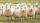There are fewer than 500 sheep, but if they stand in a double, triple, quadruple, five, and sixth-order, one sheep will remain. But they can stand in the seventh order. How many are sheep?